Dersleri yüzünden oldukça stresli bir ruh haline sikiş hikayeleri bürünüp özel matematik dersinden önce rahatlayabilmek için amatör pornolar kendisini yatak odasına kapatan genç adam telefonundan porno resimleri açtığı porno filmini keyifle seyir ederek yatağını mobil porno okşar ruh dinlendirici olduğunu iddia ettikleri özel sex resim bir masaj salonunda çalışan genç masör hem sağlık hem de huzur sikiş için gelip masaj yaptıracak olan kadını gördüğünde porn nutku tutulur tüm gün boyu seksi lezbiyenleri sikiş dikizleyerek onları en savunmasız anlarında fotoğraflayan azılı erkek lavaboya geçerek fotoğraflara bakıp koca yarağını keyifle okşamaya başlar

GET THE APP

Interference Effects of Excess Lithium on the Emission Signal of the Calcium and Strontium Ionic Lines during Inductively Coupled Plasma Emission Spectrometry: Simulation in Terms of the Simplified Collisional Radiative Recombination Rate Model
Journal of Analytical & Bioanalytical Techniques

Like us on:

# Interference Effects of Excess Lithium on the Emission Signal of the Calcium and Strontium Ionic Lines during Inductively Coupled Plasma Emission Spectrometry: Simulation in Terms of the Simplified Collisional Radiative Recombination Rate Model

Mark Fungayi Zaranyika1* and Courtie Mahamadi2

1Professor, Chemistry Department, University of Zimbabwe, Zimbabwe

2Chemistry Department, Bindura University of Science Education, Zimbabwe

*Corresponding Author:
Mark Fungayi Zaranyika
Chemistry Department
University of Zimbabwe
P. O. Box MP 167 Mount Pleasant
Harare, Zimbabwe
Tel: 263-4-303211Ext.15051
E-mail: Zaranyika@science.uz.ac.zw

Received date: March 31, 2014; Accepted date: April 29, 2014; Published date: May 01, 2014

Citation: Zaranyika MF, Mahamadi C (2014) Interference Effects of Excess Lithium on the Emission Signal of the Calcium and Strontium Ionic Lines during Inductively Coupled Plasma Emission Spectrometry: Simulation in Terms of the Simplified Collisional Radiative Recombination Rate Model. J Anal Bioanal Tech 5:190 doi: 10.4172/2155-9872.1000190

Copyright: © 2014 Zaranyika MF, et al. This is an open-access article distributed under the terms of the Creative Commons Attribution License, which permits unrestricted use, distribution, and reproduction in any medium, provided the original author and source are credited.

Visit for more related articles at Journal of Analytical & Bioanalytical Techniques

#### Abstract

ICP-AES Ca and Sr ion line signals were measured on 0-30 μg/mL Ca and Sr solutions, in the absence and presence of 1000 μg/mL Li as easily ionizable interferent, and the effect of the interferent on analyte calibration curve simulated using a simplified collisional radiative recombination rate model. Close agreement between experiment and theory was obtained for the full range of concentrations studied in the case of SrII, while the theoretical Ca ion line calibration curve exhibited close agreement below 10 μg/mL Ca concentration in the test solution, and a positive deviation of up to 18% from the experimental curve at higher Ca concentrations. The data obtained successfully demonstrates the potential of the simplified collisional radiative recombination rate model for simulating the effects of easily ionizable interferents on high ionization potential analytes.

#### Keywords

Inductively coupled plasma atomic emission spectrometry; Interference effects; Easily ionizable elements; Simplified rate model; Collisional radiative recombination; Calcium; Strontium

#### Introduction

The enhancement of emission lines from the analyte during inductively coupled plasma atomic spectrometry as a result of matrix effects has been reported by several workers [1-6]. A major objective of plasma diagnostics is to be able to predict or simulate the effects of interferents on analyte signal during such atomic spectrometric measurements. Recently we showed that the interference effects of easily ionizable elements, EIEs, on analyte absorbance or emission signal is given by Equation 1 [7-9]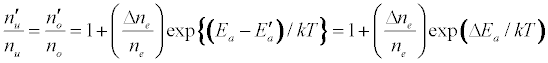Where n denotes number density, the subscripts u and o denote analyte excited state and ground state respectively, the subscript e denotes electron, Ea denotes electron activation energy, the prime denotes quantities measured in the presence of the interferent, Δne denotes the change in the electron number density upon the introduction of the interferent, k is the Boltzmann constant and T is the absolute temperature.

the absolute temperature. It can be shown on the basis of the Boltzmann population distribution law for bound states that [9,10]: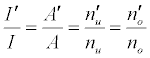where I and A denote emission and absorbance signal intensity respectively, and the prime denotes quantities measured in the presence of the interferent as before. Substitution into Equation 1 leads to Equations 3 and 4 which can be used to predict or simulate the effects of EIE interferents on analyte calibration curve during atomic absorption and emission spectrometry respectively: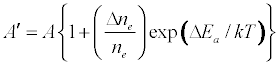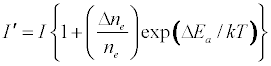Equation 1 assumes that the effects of interferents on analyte signal arise from analyte ion – electron collisional radiative recombination [7-9,11]. Current theory of atomic spectrometry assumes local thermal equilibrium (LTE), which in turn assumes that, upon ionization, electrons are ejected with kinetic energy equal to the ionization potential of the parent atom or ion, but quickly loose this energy through thermal collisions so that at thermal equilibrium all the electrons will have kinetic energy equal to kTe, whereTe is the electron temperature corresponding to LTE conditions. Zaranyika and co-workers have shown that under the conditions of LTE, ΔEa in Equations 1, 3 and 4 is equal to zero. Experimentally ΔEa has been shown to be non-zero [7-9,11], and that during air-acetylene flame and ICP atomic spectrometry analyte ion – electron collisions occur from the ambipolar diffusion state or the LTE state, so that ΔEa is given by Equations 5 and 6 respectively: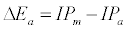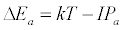where IPm and IPa denote interferent and analyte ionization potential respectively

In a previous paper  we demonstrated that the Mg emission calibration curve, determined in the presence of excess Ca, Ba and Sr using air-acetylene flame atomic emission spectrometry, can be simulated with close agreement between theory and experiment using Equations 3 and 5. In this communication we report the results obtained when Equations 4 and 6 are used to simulate the effect of excess Li on the ICP-AES calibration curve for CaII and SrII. The interference effects of excess Li on CaI and SrII have been simulated before with good agreement between theory and experiment using a simplified rate model based on charge transfer between analyte ions and excited interferent atoms . The charge transfer rate model differs from the collisional radiative recombination rate model in that the charge transfer rate model assumes charge transfer involving interferent electrons in bound excited states, whereas the radiative recombination rate model assumes collisions involving interferent electrons in the continuum state. The short-comings of the charge transfer rate model have been discussed previously , and include the fact that the charge transfer rate model is not able to explain the non-involvement of Ar species in the collisional processes leading to the observed interference effects.

#### Experimental

ICP equipment

A Unicam 701-Emission Inductively Coupled Argon Plasma Echelle Spectrophotometer with “crossed” dispersion was used. The spectrophotometer was fitted with an aperture plate of 90 mm by 1.5 nm slits etched at 2 mm intervals, a photomultiplier tube (PMT) detector mounted on a movable frame for radial view of the ICP, a torch with three concentric tubes for outer gas, auxiliary gas and sample transport, a 40.68 MHz R.F. generator which supplies power up to 2 kW, a grid type nebulizer fitted with Pt screens and Pt orifice, a 5-channel computer controlled peristaltic pump, and an automatic Ar gas flow rate optimizer.

The argon (99.95%) was supplied from a pressurised tank (BOC GAS Ltd, Zimbabwe): The outer gas was supplied at 14 L min-1, power 1.0 kW, nebulizer pressure 40 psi (280 kPa) and auxiliary gas at 1 L min-1. Under these conditions the plasma temperature is between 7000 and 9000 K . The optimum zone for analysis was established using the strong emission line of Mn, and the maximum temperature of 9000 K was used in the calculations for maximum effect of the interferent. CaII and SrII line emission signals were recorded at 393.3 and 407.8 nm respectively.

Materials

The following were used: Calcium chloride dehydrate, M = 147.02 g/mol., AR grade (impurities present: sulphate 0.005%, total nitrogen 0.005%, phosphorous 0.001%, lead 0.001%, iron 0.0005%, magnesium 0.01%, sodium 0.01% and potassium 0.01%); Strontium chloride hexahydrate, M = 266.62 g/mol., AR grade (impurities: water insoluble matter 0.005%, sulphate 0.001%, lead 0.0002%, iron 0.0001%, zinc 0.0001%, barium 0.02%, and substances not precipitated by sulphuric acid 0.0002%); Lithium Chloride, M = 42.39 g/mol., Merck GR grade (impurities: sulphate 0.005%, total nitrogen 0.001%, heavy metals ( as lead) 0.0005%, iron 0.0005%, calcium (Ca) 0.005%, magnesium (Mg) 0.005%, sodium (Na) 0.002% and potassium (K) 0.02%) (Merck, Darmstadt, Germany); Deionised water of conductivity 0.001 μSm-1.

Procedure

The experimental procedure was described previously [7,8]. Two sets of standard solutions containing 0 to 30.0 mg/L analyte (Sr or Ca) were prepared from freshly prepared solutions of their chloride salts. One set was spiked with 1000 mg/L of LiCl as EIE interferent. The other set was left unspiked. The experiment was designed such that the interferent concentration was kept constant at a very high level (1000 mg/L) relative to that of the analyte, whilst the analyte concentration was varied. In this way changes in the physical properties of the test solution upon the introduction of the interferent could be minimized, and be compensated for by taking blank readings of a solution containing the interferent salt only. Preliminary experiments were run to determine the aspiration rate and the nebulization efficiency for the type of solutions under analysis [10,14,15]. Mean values obtained for the aspiration rate and nebulization efficiency were 1.00 ± 0.04 L/min and 5.0 ± 0.7% (n = 8) respectively

Analyte line emission signals, I and I’ where the prime denotes presence of the interferent, were read in triplicate. Typical I and I’ results obtained are shown in Table 1. The values of I’ for Ca were corrected for the contribution from contamination from the interferent, and plotted as a function of analyte concentration in the test solution in Figure 1.

c* CaII SrII
[Li]= 0 mg/L [Li]= 1000 mg/L [Li]= 0 mg/L [Li] = 1000 mg/L
0.0 142250 ± 1588 126216 ± 921
0.1     877 ± 11 1983 ±13
0.2 778553 ± 6645 2081694 ± 1484 1178 ± 10 2179 ± 9
0.4 1235614 ± 1237 2689027 ± 6978 2182 ± 33 3033 ± 35
0.6 1908893 ± 2111 3626017 ± 1882 3590 ± 58 4488 ± 52
0.8 2721784 ± 4162 4079534 ± 2820 5651 ± 46 6679 ± 43
2.0 8306215 ± 7933 8355747 ± 1425 9976 ± 122 11482 ± 119
4.0 14615291 ± 5822 14617597 ± 579 13388 ± 253 14606 ± 250
8.0 32097324 ± 1829 29707464 ± 2815 20044 ± 232 20986 ± 256
10.0 33203770 ± 1859 33214872 ± 4407 26421 ± 324 27399 ± 336
12.0     34207 ± 315 34789 ± 345
14.0 53349413 ± 1126 49002586 ± 2537
16.0     37960 ± 456 38909 ± 412
20.0 78537595 ± 1057 67945785 ± 5195 41483 ± 513 42271 ± 522
30.0 120000000 ± 1566 97605488 ± 3163 47523 ± 815 49376 ± 789

Table 1: I and I’ values: Effect of excess Li interferent on CaII line (λ = 393.6 nm) and SrII line (λ = 407.8 nm) (n =3).

Theoretical calculations

Analyte and interferent ion number densities were calculated assuming the aspiration rate and nebulization efficiency values obtained above and 9000 K temperature. Sr+ and Ca+ number densities assumed 99.40% and 98.90% degree of ionization respectively , while those for Sr2+ and Ca2+ assumed 19.72% and 6.43% degree of ionization respectively . The number density of Li+ ions was calculated using the Saha equation :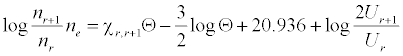where nr and nr+1 are the number densities of atoms in the r and r+1 stages of ionization, Ne is the number density of electrons, χr, r+1 is the ionization potential in eV from the r to the r+1 stage of ionization, Θ = 5040°K/T (T = plasma temperature), Ur and Ur+1 are the partition coefficients, and the factor 2 represents the statistical weight of an electron. Number densities obtained are shown is Table 2 .

M* Flow number density (atoms/ions cm-3s-1)
MI MII MIII
Ca 2.5147 ×1010 c** 2.46 ×1010c 1.5818 ×109 c
Sr 1.1377 ×1010 c 1.1309 ×1010 c 2.2301 ×109 c
Ar 2.6883 ×1019 2.9242 ×1016
Li 1.4367 ×1014 1.4367 ×1014

Table 2: Analyte and interferent atom and ion number densities in the ICP (T =9000°C, 1.0 L.min-1, and 5.0% nebulization efficiency).

#### Results and Discussion

Substitution of the appropriate ionization potentials into Equation 6 yields the ΔEa values in Table 3 for CaII/Li and SrII/Li analyte/interferent systems. Substitution of the appropriate values from Tables 2 and 3 into Equation 4 yields the equations for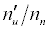in Table 3.

Analyte IPa (eV)a Interferent IPm(eV)a ΔEa (eV)Equationb
CaII 11.868 Li 5.39172 -11.092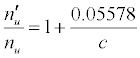SrII 11.03013 Li 5.39172 -10.255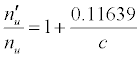Table 3: ΔEa values and u n n′ n equations for CaII and SrII in the ICP.

Assuming Equation 4 holds, then the emission signals in the presence of the interferent for the CaII/Li and SrII/Li analyte/interferent systems are given Equations 8 and 9 respectively: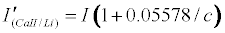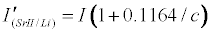Theoretical calibration curves predicted on the basis of Eqs 8 and 9 for the effects of 1000 mg/L excess Li on CaII and SrII line emission signals in the ICP are shown in Figure 1, together with the experimental I’/I curves for comparison. It is apparent from Figure 1 that for SrII good agreement between the theoretical and experimental calibration curves is obtained throughout the range of Sr concentration studied. For CaII, good agreement is also obtained, although a negative deviation of the experimental calibration curve from the theoretical curve of up to about 18% is obtained above 10 mg/L Sr concentration in the test solution. This negative deviation of the experimental curve could be due to a drift in the plasma temperature during the course of the determinations. Errors due to system drift have been reported by Cheung et al. . Otherwise, a deviation of 18% can be considered acceptable experimental error for the type of experiments under consideration.

Figure 1: Experimental and theoretical calibration curves when (a) CaII and (b) SrII is determined by ICP-AES in the presence of excess Li interferent (Expt = experimental; Theo/theory = theoretical).

The agreement between experiment and theory as shown in Figure 1 confirm the assumptions made in arriving at Equation 4, namely (a) that Ar species and electrons from the ionization of Ar are not involved in collisional processes leading to the observed interference effects when analytes are determined by ICP-AES in the presence of easily ionizable interferents, and (b) that collisional radiative recombination is the major collisional process leading to analyte excited state, and hence the observed interference effects [7,8]. The results obtained correspond to pre-LTE collisional radiative recombination in the absence of the interferent, and LTE collisional radiative recombination in the presence of the interferent [7-9], i.e., electronic collisions involving electrons from the ionization of the analyte occur from the ambipolar diffusion state, while collisions involving electron from the ionization of the EIE interferent occur from the LTE state after thermal equilibration. The fact that electrons from the ionization of Ar are not involved in the collisional processes leading to observed interference effects, can only be explained by assuming that collisions with these electrons occur while the electrons are still in the ambipolar diffusion layer, when their kinetic energy at 15.755 eV (i.e., the ionization potential of Ar) is far in excess of that required for radiative recombination with CaII and SrII ions. Such collisions must be elastic, otherwise the effects of collisional radiative recombination involving electrons from the ionization of the interferent, at much lower concentration in the plasma, would not be observed. The results also confirm our earlier observation [7-9] that the lifetime of electrons in the ambipolar diffusion layer appears to be inversely related to the ionization potential of the parent atom or ion. A kinetic model to account for this observation has been proposed . Further work is underway in our laboratories to test the applicability of Equation 4 to other analyte/interferent systems.

#### Conclusion

From the foregoing discussion we conclude that interference effects due to easily ionizable elements on analyte line emission signal during ICP-AES can be simulated successfully using the simplified rate model based on collisional radiative recombination as the major collisional process leading to the observed interference effects. Although the work presented in this paper is rather limited in scope, the remarkable success of the model in predicting the interference of excess Li on the emission signal of SrII, confirms the potential of the model in simulating analyte emission signal enhancement interference effects during ICP-AES. The results presented constitute further confirmation that the ICP is not in LTE, and suggest that electrons from the ionization of easily ionizable elements exist primarily in the LTE state, while those from the high ionization potential analytes and Ar exist primarily in the ambipolar diffusion state.

#### Acknowledgements

Financial support for this work was provided by a grant from the Research Board of the University of Zimbabwe (UZ). The assistance of the Director and staff of the Institute of Mining Engineering, UZ, and the management and staff of Bindura Nickel Corporation, Bindura, Zimbabwe, in availing us the use of their ICP equipment is gratefully acknowledged.

--

### Article Usage

• Total views: 14053
• [From(publication date):
July-2014 - Sep 26, 2023]
• Breakdown by view type
• HTML page views : 9686Can't read the image? click here to refresh